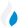•Trade Pi
•Trade
•Exchange
•Trade Pi
•Trade
•Exchange
\$1.12T
Total marketcap
\$73.63B
Total volume
39.42%
BTC dominance
•Trade Pi
•Trade
•Exchange

# amp to INR Exchange Rate - 1 Amp in INR

0.81812214423507
• BTC 0.00000022
• ETH 0.000003
Vol [24h]
\$16,977.09

## amp to INR converter

Exchange Pair Price 24h volume
ZebPay AMP/INR \$0.01161 \$14.46K
WazirX AMP/INR \$0.005072 \$2.29K

## AMP/INR Exchange Rate Overview

Name Ticker Price % 24h 24h high 24h low 24h volume
Amp amp \$0.004983 -3.2817% \$0.005262 \$0.004796 \$9.16M

Selling 1 Amp amp you get 0.81812214423507 INR.

Amp Jun 16, 2021 had the highest price, at that time trading at its all-time high of \$0.1208.

603 days have passed since then, and now the price is 4.09% of the maximum.

Based on the table data, the AMP vs INR exchange volume is \$16,977.09.

Using the calculator/converter on this page, you can make the necessary calculations with a pair of Amp to INR.

## Q&A

### What is the current AMP to INR exchange rate?

Right now, the AMP/INR exchange rate is 0.81812214423507.

### What has been the Amp to INR trading volume in the last 24 hours?

Relying on the table data, the Amp to INR exchange volume is \$16,977.09.

### How can I calculate the amount of INR? / How do I convert my Amp to INR?

You can calculate/convert INR from AMP to INR converter. Also, you can select other currencies from the drop-down list.

## AMP to INR Сonversion Table

AMP INR
200 AMP = 163.6 INR
300 AMP = 245.4 INR
500 AMP = 409.06 INR
700 AMP = 572.6 INR
2000 AMP = 1,636.2 INR
3000 AMP = 2,454.3 INR
4000 AMP = 3,272.4 INR
20000 AMP = 16,362.4 INR
30000 AMP = 24,543.6 INR
300000 AMP = 245,436.6 INR
3000000 AMP = 2,454,366.4 INR
30000000 AMP = 24,543,664.3 INR
300000000 AMP = 245,436,643.2 INR